# Heat maps: autoimage and ggplot2

## Introduction

The autoimage package makes it easy to plot a sequence of images with corresponding color scales, i.e., a sequence of heatmaps, with straightforward, native options for projection of geographical coordinates. The package makes it simple to add lines, points, and other features to the images, even when the coordinates are projected. The package allows for seamless creation of heat maps for data on regular or irregular grids, as well as data that is not on a grid.

The well-known ggplot2 is a powerful and complete system for producing graphics in R. It can be used to produce many of the same heat maps as the autoimage package, particularly if additional packages are loaded.

In what follows, we look at how these packages can be used to produce various heat maps.

## Examples

In what follows, we make use of data from the North American Regional Climate Change Assessment Program (NARCCAP, http://www.narccap.ucar.edu/). Specifically, the data are the maximum daily surface air temperature (K) (abbreviated tasmax) for the five consecutive days of May 15, 2041 to May 19, 2041 simulated using the Canadian Regional Climate Model (Caya and Laprise, 1999) forced by the Community Climate System Model atmosphere-ocean general circular model (Collins et al., 2006). The data set contains lon, a 140 $$\times$$ 115 matrix of longitude coordinates, lat, a 140 $$\times$$ 115 matrix of latitude coordinates, and tasmax, a 140 $$\times$$ 115 $$\times$$ 5 array, where each element of the third dimension of the array corresponds to the tasmax measurements of the respective day.

### Basic Sequence of Heat Maps

We begin by creating a basic heat map using the two packages.

As a preliminary, we create a day vector related to the date of the temperatures.

data(narccap)
dates <- c("May 15, 2041", "May 16, 2041", "May 17, 2041", "May 18, 2041", "May 19, 2041")

We produce a sequence of temperature heat maps using autoimage, labeling each image with the corresponding day.

autoimage(x = lon, y = lat, z = tasmax, main = dates)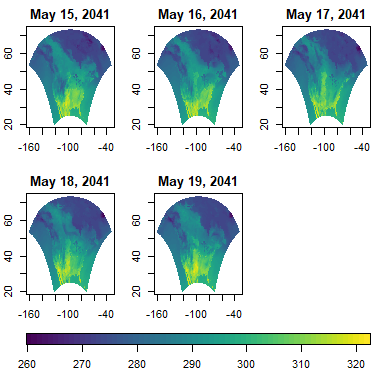To produce a similar plot using the ggplot2 package, we must convert the data to a data frame, create a ggplot object, add the tile geometry, and facet by day. However, ggplot2 currently requires the data are on a regular grid, which are data not. If we attempt to create a heat map for a small subset of the original data using ggplot2, we obtain the following result. Because the original data are on an irregular grid, ggplot2 cannot (currently) render a heat map for the data.

df1 <- data.frame(lon = c(lon[65:75, 50:60]), lat = c(lat[65:75, 50:60]),
tasmax = c(tasmax[65:75, 50:60, 1]))
ggplot(df1, aes(x = lon, y = lat, z = tasmax, fill = tasmax)) + geom_tile()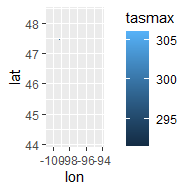Consequently, we load the inarccap data, which is the narccap data interplated onto a regular 140 $$\times$$ 115 grid using the interp function in the akima package.

data(inarccap)

We now convert the interpolated data to a data frame suitable for ggplot2.

igrid <- expand.grid(ilon, ilat)
df <- data.frame(lon = igrid[,1], lat = igrid[,2],
tasmax = c(itasmax),
day = rep(dates, each = 16100)) 

We are now able to create a sequence of heat maps using the ggplot2 package.

ggplot(df, aes(x = lon, y = lat, fill = tasmax)) + geom_tile() +
facet_wrap(~ day)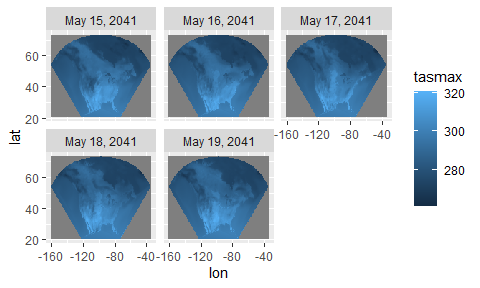To create a more user-friendly color palette, we use the scale_fill_viridis function in the viridis package. The grey shading (associated with NA values) can be removed by specifying na.value = "transparent" in scale_fill_viridis. We remove the solid grey grid background by using the theme_bw function. Lastly, we change the orientation and location of the color scale by setting the legend.position argument of theme.

library(viridis)
## Loading required package: viridisLite
ggplot(df, aes(x = lon, y = lat, fill = tasmax)) +
geom_tile() + facet_wrap(~ day) + scale_fill_viridis(na.value = "transparent") +
theme_bw() + theme(legend.position = "bottom")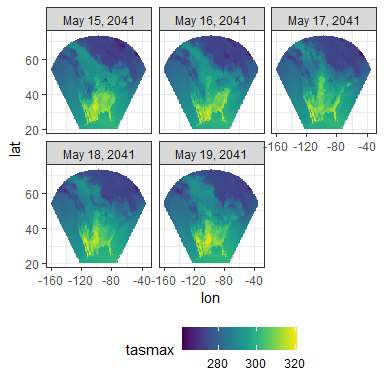### Coordinate Projection

The locations of data used to create heat maps are often measured in longitude and latitude coordinates. In that case, it is often appropriate to apply a projection to the coordinates before plotting. Both autoimage and ggplot2 provide native functionality for create heat maps for projected coordinates. This is done by specifying the proj and parameters arguments in the autoimage function for the autoimage package, or specifying the arguments of the coord_map function for ggplot2.

We demonstrate how to do this below by adding a specific Bonne projection to the previous examples.

autoimage(x = lon, y = lat, z = tasmax, main = dates,
proj = "bonne", parameters = 45)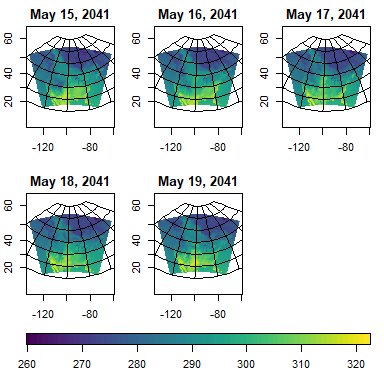Rendering the heat maps with a projection takes noticeably longer when using the ggplot2 package in comparison with the autoimage package, though exact timings depend on the user’s computer. For efficiency reasons, we do not execute the command below, though doing so would produce a heat map with projected coordinates using ggplot2.

# ggplot(df, aes(x = lon, y = lat, fill = tasmax)) + geom_tile() +
#   facet_wrap(~ day) + scale_fill_viridis() + theme_bw() +
#   coord_map(projection = "bonne", parameters = 45) +
#   theme(legend.position = "bottom")

It is sometimes helpful to add geographical features of interest to a heat map, e.g., provincial borders, city locations, etc. This can be done using either package.

Both packages have several geographic maps built into the software. The autoimage package can automatically pull a map directly from the maps by specifying the appropriate name to the map argument. ggplot2 has the same maps, which can be accessed using the map_data function. The map (specifically, the map boundaries) can then be added to the heat map using the geom_path function. If the map borders extend beyond the range of observed data, then the x and y limits must be provided to the ggplot object.

autoimage(x = lon, y = lat, z = tasmax, main = dates, map = "world")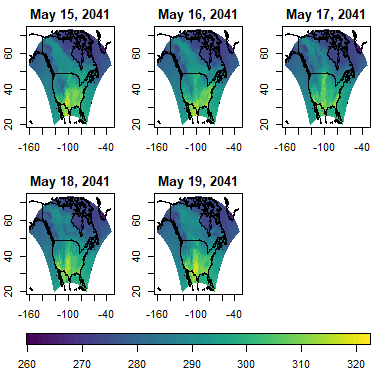world_df <- map_data("world")
ggplot() + geom_tile(aes(x = lon, y = lat, fill = tasmax), data = df) +
facet_wrap(~ day) + scale_fill_viridis() + theme_bw() +
geom_path(aes(x = long, y = lat, group = group), data = world_df) +
xlim(c(-160, -34)) + ylim(c(20.5, 73.1)) +
theme(legend.position = "bottom")
## Warning: Removed 410171 row(s) containing missing values (geom_path).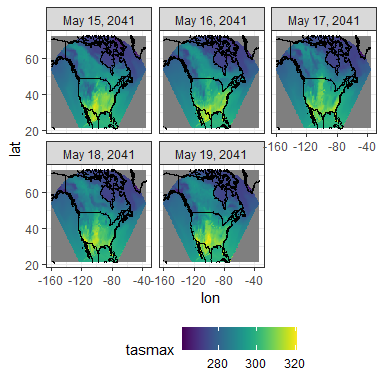Consider arbitrary maps available in a standard shapefile. The readOGR and spTransform functions in the rgdal package can be used to read the shapefile and convert it to a SpatialPolygon object, which is used in the sp package. The SpatialPolygons2map function in the maps package can then be used to convert the object into a format used by the maps package. The autoimage package uses the same format for its maps and lines. These objects must be converted to a data frame before use with the ggplot2 package.

Consider the canada data in the autoimage package, which contains the provincial and territorial boundaries for Canada. The data are already in the map format utilized by the maps and autoimage packages. We can convert this to a data frame compatible with ggplot2 using the tidy function in the broom package.

data(canada)
library(broom)
canada_df <- tidy(canada)

We now add the desired boundaries to the heat maps using each package.

autoimage(x = lon, y = lat, z = tasmax, main = dates, lines = canada)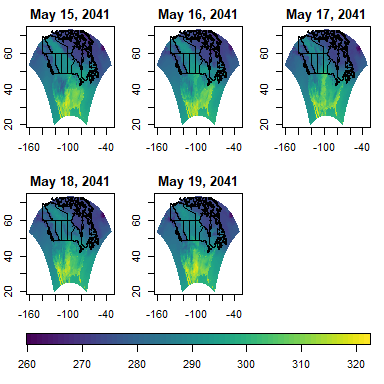ggplot() + geom_tile(aes(x = lon, y = lat, fill = tasmax), data = df) +
facet_wrap(~ day) + scale_fill_viridis() +
geom_path(aes(x = long, y = lat, group = group), data = canada_df) +
theme_bw() + theme(legend.position = "bottom")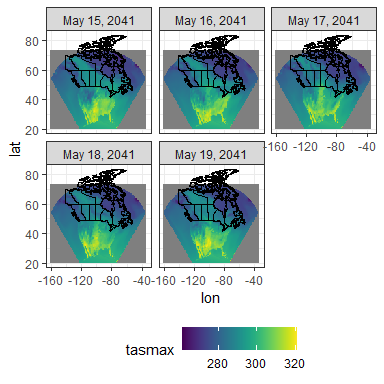Next, we consider adding points to existing heat maps. We plot a subset of the us.cities data found in the maps package. The autoimage is expecting the data frame to have components x and y specifying the x and y coordinates of the locations, so we create a data frame with this structure before plotting. The same data frame can be used by ggplot2.

caps <- maps::us.cities[maps::us.cities$capital == 2, ] caps <- caps[c(1, 3, 5, 22, 27, 42), ] cap_df <- data.frame(x = caps$lon, y = caps$lat, labels = caps$country.etc)

We now create the desired heat maps.

autoimage(x = lon, y = lat, z = tasmax, main = dates, text = cap_df)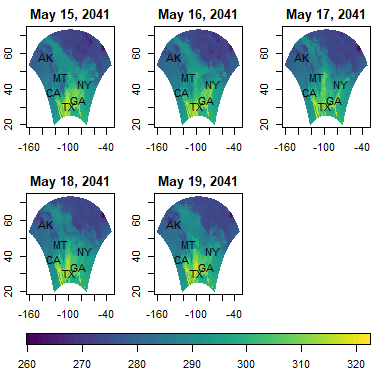ggplot() + geom_tile(aes(x = lon, y = lat, fill = tasmax), data = df) +
facet_wrap(~ day) + scale_fill_viridis() +
geom_text(aes(x = x, y = y, label = labels), data = cap_df) +
theme_bw() + theme(legend.position = "bottom")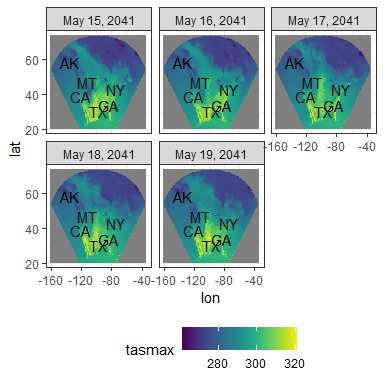## Individual heat maps

Sometimes there are reasons to construct heat maps with individual color scales, e.g., when the data are on different scales such as heat maps of predicted value versus standard error. This can be done natively in autoimage, but requires loading the gridExtra package for ggplot2. We demonstrate this functionality by creating heat maps with individual color scales for each day of the narccap data.

To create a sequence of images with individual color scales using the autoimage package, one simply specifies common.legend = FALSE.

autoimage(lon, lat, tasmax, common.legend = FALSE, main = dates)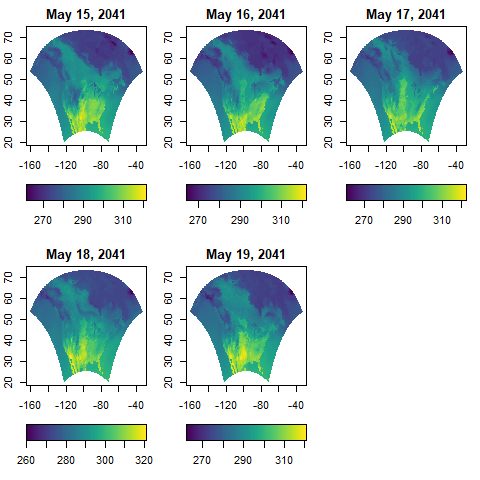To obtain a similar result using ggplot2, we first split the df data frame into a list of data frames, with each element of the list corresponding to the data for one of the days. We then create heat maps of the data for each day, then combine them into one plot using the grid.arrange function in the gridExtra package.

df_days = split(df, f = df\$day)
p1 <- ggplot(df_days[], aes(x = lon, y = lat, fill = tasmax)) +
geom_tile() + labs(title = dates) +
theme(legend.position = "bottom")
p2 <- ggplot(df_days[], aes(x = lon, y = lat, fill = tasmax)) +
geom_tile() + labs(title = dates) +
theme(legend.position = "bottom")
p3 <- ggplot(df_days[], aes(x = lon, y = lat, fill = tasmax)) +
geom_tile() + labs(title = dates) +
theme(legend.position = "bottom")
p4 <- ggplot(df_days[], aes(x = lon, y = lat, fill = tasmax)) +
geom_tile() + labs(title = dates) +
theme(legend.position = "bottom")
p5 <- ggplot(df_days[], aes(x = lon, y = lat, fill = tasmax)) +
geom_tile() + labs(title = dates) +
theme(legend.position = "bottom")
library(gridExtra)
grid.arrange(p1, p2, p3, p4, p5, nrow = 2, ncol = 3)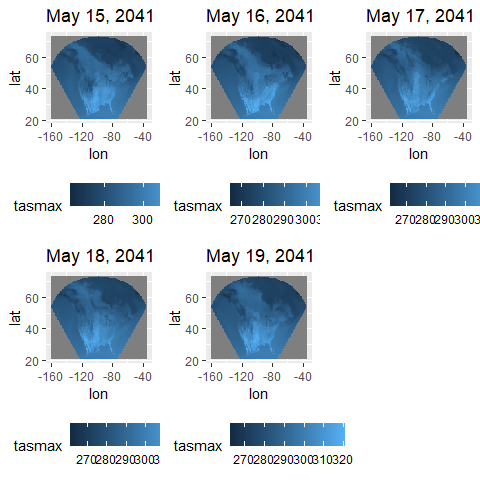## Conclusion

In this vignette, we have briefly compared the approaches for producing heat maps using the autoimage and ggplot2 packages. The packages have fairly similar capabilities for producing heat maps, though the style and steps to obtain the heat maps sometimes differ considerably. Additionally, the ggplot2 package can be noticeably slower when producing heat maps for projected coordinates.